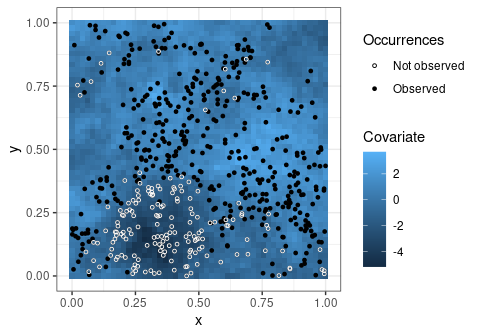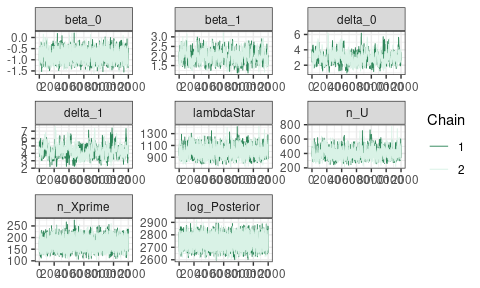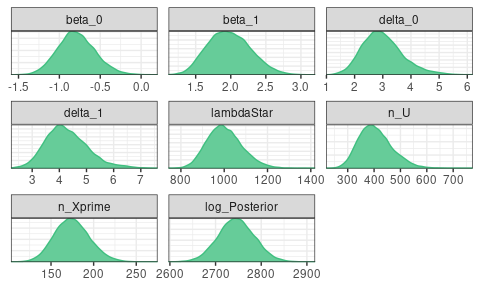# bayesPO

library(bayesPO)
library(ggplot2)
library(bayesplot)
#> This is bayesplot version 1.10.0
#> - Online documentation and vignettes at mc-stan.org/bayesplot
#> - bayesplot theme set to bayesplot::theme_default()
#>    * Does _not_ affect other ggplot2 plots
#>    * See ?bayesplot_theme_set for details on theme setting
library(MASS)
theme_set(theme_bw())
color_scheme_set("green")
set.seed(123456789)
temp <- tempfile(fileext = ".rda")
temp, mode = "wb", quiet = TRUE)
# Try to use downloaded version. If not available, run the model
if (!d) load(temp, verbose = TRUE) else {
}
#>   Z
#>   occurrences
#>   W
#>   po_sightings
#>   fit
#>   fit2

In this vignette, we show the basics of fitting a model with the bayesPO package. For this purpose we use some simulated data.

## Simulating some data

We simulate some simple data from the model in the unit square to showcase it being used. First we set some true values for the parameters.

if (!d) {
beta <- c(-1, 2) # Intercept = -1. Only one covariate
delta <- c(3, 4) # Intercept = 3. Only one covariate
lambdaStar <- 1000
} else warning("Data failed to download from drive. Please check internet connection and try again.")

The code below just does this simulation.

if (!d) {
# Spread a Poisson amount of points randomly in the random square.
total_points <- rpois(1, lambdaStar)
random_points <- cbind(runif(total_points), runif(total_points))
grid_size <- 50

# Find covariate values to explain the species occurrence.
# We give them a Gaussian spatial structure.
reg_grid <- as.matrix(expand.grid(seq(0, 1, len = grid_size), seq(0, 1, len = grid_size)))
#### Next is commented to save time. Uncomment to replicate results
# Z <- mvrnorm(1, rep(0, total_points + grid_size * grid_size), 3 * exp(-as.matrix(dist(rbind(random_points, reg_grid))) / 0.2))
Z1 <- Z[1:total_points]; Z2 <- Z[-(1:total_points)]

# Thin the points by comparing the retaining probabilities with uniforms
# in the log scale to find the occurrences
#### Next is commented to save time. Uncomment to replicate results
# occurrences <- log(runif(total_points)) <= -log1p(exp(-beta - beta * Z1))
n_occurrences <- sum(occurrences)
occurrences_points <- random_points[occurrences,]
occurrences_Z <- Z1[occurrences]

# Find covariate values to explain the observation bias.
# Additionally create a regular grid to plot the covariate later.
#### Next is commented to save time. Uncomment to replicate results
# W <- mvrnorm(1, rep(0, n_occurrences + grid_size * grid_size), 2 * exp(-as.matrix(dist(rbind(occurrences_points, reg_grid))) / 0.3))
W1 <- W[1:n_occurrences]; W2 <- W[-(1:n_occurrences)]

# Find the presence-only observations.
#### Next is commented to save time. Uncomment to replicate results
# po_sightings <- log(runif(n_occurrences)) <= -log1p(exp(-delta - delta * W1))
n_po <- sum(po_sightings)
po_points <- occurrences_points[po_sightings, ]
po_Z <- occurrences_Z[po_sightings]
po_W <- W1[po_sightings]
} else warning("Data failed to download from drive. Please check internet connection and try again.")

Now the variable po_points contains the coordinates of the presence-only sightings. We can plot the observability covariate to see how the points have been selected and discarded according to it.

if (!d) {
ggplot(
data.frame(x = reg_grid[, 1], y = reg_grid[, 2], Covariate = W2),
aes(x, y)
) +
geom_tile(aes(fill = Covariate)) +
geom_point(aes(shape = Occurrences),
data = data.frame(x = occurrences_points[, 1],
y = occurrences_points[, 2],
Occurrences = po_sightings), size = 1) +
geom_point(data = data.frame(x = occurrences_points[!po_sightings, 1],
y = occurrences_points[!po_sightings, 2]),
size = 1, shape = 1, color = "white") +
scale_shape_manual(values = c(1, 19), labels = c("Not observed", "Observed"))
} else warning("Data failed to download from drive. Please check internet connection and try again.")The points are more often observed when the covariate is large, as it should. Note that there is a spatial pattern to all occurrences, and it is caused by the intensity covariate’s pattern (not plotted).

## Creating the model

In the bayesPO package, the model is built separately, so that it can be saved to disk and it can be easily recovered. It must contain the data, meaning the matrix with the covariates in the observed locations, the selection of covariates, the link function (although for now only the logit link is supported), initial values for the Markov Chain and the prior.

First we create a prior distribution. Currently, only a Normal prior is accepted for the Beta and Delta parameters, and only a Gamma prior is accepted for the LambdaStar parameter.

if (!d) {
jointPrior <- prior(
NormalPrior(rep(0, 2), 10 * diag(2)), # Beta
NormalPrior(rep(0, 2), 10 * diag(2)), # Delta
GammaPrior(0.00001, 0.00001) # LambdaStar
)
} else warning("Data failed to download from drive. Please check internet connection and try again.")

The prior is set with expected value 1 and very high variance for LambdaStar, but not that high variance for Beta and Delta. The reason for the former is that it is very hard to interpret and elicit reasonable values for this parameter, but it should be as small as possible (larger values yield more calculations and a slower program).

The choice for the Beta and Delta prior is twofold. Firstly, all covariates should be standardized for stability in the logistic scale, and the values for these parameters should not be very large (in absolute value), for the same reason. Secondly, a smaller variance provides some regularization, which is always desired in regression problems.

The next step is creating the model.

if (!d) {
model <- bayesPO_model(po = cbind(po_Z, po_W),
intensitySelection = 1, observabilitySelection = 2,
initial_values = 2, joint_prior = jointPrior)
#> Data loaded successfully with 1 intensity variables and 1 observability variables selected.
#> 2 chains were initialized.
#> Intensity covariates selected with column indexes. Make sure the background covariates are in the same position.
#> Observability covariates selected with column indexes. Make sure the background covariates are in the same position.

The instruction initial_values = 2 informs the model that it is supposed to run two chains, where the initial values are randomly generated.

## Fitting the model

Only one piece is needed for the model, namely the matrix with the background variables. Note that this is usually a very large matrix, which is why it is not included in the model, that is, to keep it a small object. Afterwards, only fitting the model remains.

if (!d) {
bkg <- cbind(Z2, W2) # Create background

#### Next is commented to save time. Uncomment to replicate results
# fit <- fit_bayesPO(model, bkg, area = 1, mcmc_setup = list(burnin = 1000, iter = 2000))

## Checking results

As with any MCMC procedure, the chains need to be checked. The first thing to do is print the fit.

if (!d) {
summary(fit)
#>                    mean      median         sd       2.5%        97.5%
#> beta_0       -0.7825216  -0.7919222  0.2318341  -1.207057   -0.3100689
#> beta_1        1.9804734   1.9553691  0.3197719   1.443432    2.6172018
#> delta_0       2.9853829   2.9168782  0.6843450   1.847569    4.4866229
#> delta_1       4.2716921   4.1766663  0.7556180   3.017082    5.8660294
#> lambdaStar 1002.2745627 994.9388105 83.5088060 859.887895 1178.2802747
#>            eff. sample size Estimated Rhat Upper CI Rhat
#> beta_0            175.10494       1.002053      1.008319
#> beta_1             56.38168       1.029380      1.058563
#> delta_0            48.25616       1.081209      1.317752
#> delta_1            33.54868       1.110962      1.412294
#> lambdaStar        129.04037       1.006387      1.031887

The Rhat columns gives some metric for quality of convergence. It is only provided when there is more than 1 fitted chain. Ideally, the upper limit CI should be below 1.1. Since this is not true for some of the parameters, more iterations should be run. This can be done by recalling the function on the last fitted object. It starts where the last one left off.

#### Next is commented to save time. Uncomment to replicate results
# fit2 <- fit_bayesPO(fit, bkg, mcmc_setup = list(iter = 10000))

Checking the new fit…

if (!d) {
summary(fit2)
#>                    mean      median         sd       2.5%        97.5%
#> beta_0       -0.7898889  -0.7999272  0.2286448  -1.215161   -0.3157861
#> beta_1        1.9799571   1.9651421  0.3018231   1.441253    2.5992603
#> delta_0       2.9988409   2.9310663  0.7196911   1.783329    4.6392346
#> delta_1       4.2836509   4.2046688  0.7818815   2.950952    6.0831205
#> lambdaStar 1003.0047876 997.2003271 81.3932604 860.104638 1177.8486214
#>            eff. sample size Estimated Rhat Upper CI Rhat
#> beta_0            1048.5189       1.001027      1.003760
#> beta_1             316.8509       1.005522      1.022658
#> delta_0            205.1215       1.006685      1.017748
#> delta_1            183.5131       1.006680      1.017484
#> lambdaStar         628.2132       1.001675      1.007639

… and it looks better. To see the traceplots, package bayesplot is very useful. It automatically calls the as.array() method, and the fitted object is readied for the bayesplot functions.

if (!d) {
mcmc_trace(fit2)
} else warning("Data failed to download from drive. Please check internet connection and try again.")Satisfied with convergence, the marginal distributions can be checked.

if (!d) {
mcmc_dens(fit2)
} else warning("Data failed to download from drive. Please check internet connection and try again.")There is high posterior density close to the parameters true values, which is good!